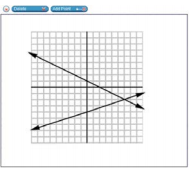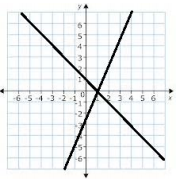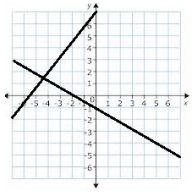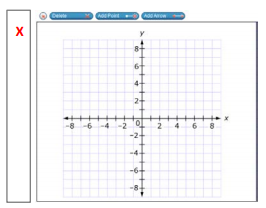# MAFS.8.EE.3.8Archived StandardExport Print
Analyze and solve pairs of simultaneous linear equations.
1. Understand that solutions to a system of two linear equations in two variables correspond to points of intersection of their graphs, because points of intersection satisfy both equations simultaneously.
2. Solve systems of two linear equations in two variables algebraically, and estimate solutions by graphing the equations. Solve simple cases by inspection. For example, 3x + 2y = 5 and 3x + 2y = 6 have no solution because 3x + 2y cannot simultaneously be 5 and 6.
3. Solve real-world and mathematical problems leading to two linear equations in two variables. For example, given coordinates for two pairs of points, determine whether the line through the first pair of points intersects the line through the second pair.

### Remarks

Examples of Opportunities for In-Depth Focus

When students work toward meeting this standard, they build on what they know about two-variable linear equations, and they enlarge the varieties of real-world and mathematical problems they can solve.
General Information
Subject Area: Mathematics
Domain-Subdomain: Expressions & Equations
Cluster: Level 2: Basic Application of Skills & Concepts
Cluster: Analyze and solve linear equations and pairs of simultaneous linear equations. (Major Cluster) -

Clusters should not be sorted from Major to Supporting and then taught in that order. To do so would strip the coherence of the mathematical ideas and miss the opportunity to enhance the major work of the grade with the supporting clusters.

Date of Last Rating: 02/14
Status: State Board Approved - Archived
Assessed: Yes
Test Item Specifications

• Assessment Limits :
Numbers in items must be rational numbers. Coefficients of equations in standard form must be integers. Items written for MAFS.8.EE.3.8a must include the graph or the equations. Equations in items written for MAFS.8.EE.3.8a must be given in slope-intercept form.
• Calculator :

Yes

• Context :

Allowable

Sample Test Items (8)
• Test Item #: Sample Item 1
• Question: A graph of a system of two equations is shown.

Use the Add Point tool to plot the solution of the system.• Difficulty: N/A
• Type: GRID: Graphic Response Item Display

• Test Item #: Sample Item 2
• Question:

Analyze the system of two equations shown.

y=3(x+4)

y=3(x-4)

How many solutions does the system of equations have?

• Difficulty: N/A
• Type: SHT: Selectable Hot Text

• Test Item #: Sample Item 3
• Question:

A graph of a system of two equations is shown.What is the solution of the system?

• Difficulty: N/A
• Type: EE: Equation Editor

• Test Item #: Sample Item 4
• Question:

A graph of a system of two equations is shown.What is the approximate solution of the system?

• Difficulty: N/A
• Type: EE: Equation Editor

• Test Item #: Sample Item 5
• Question:

A system of two equations is shown.

y = 5x + 3

y = 3x - 1

A. Use the Add Arrow tool to graph the two lines.

B. Drag the palette image to show the solution of the system.• Difficulty: N/A
• Type: GRID: Graphic Response Item Display

• Test Item #: Sample Item 6
• Question: Radha is trying to choose between two bike rental companies, Company A and Company B.

Company A charges a \$25 initial fee and an additional \$5 for each hour rented. Company B charges an initial \$18 fee and an additional \$6 for each hour rented.

The total cost to rent a bike from Company A can be represented by the equation y=5h+25, where h represents the number of hours rented and y represents the cost, in dollars.

The total cost to rent a bike from Company B can be represented by the equation y=6h+18, where h represents the number of hours rented and y represents the cost, in dollars.

For how many hours of rental is the amount charged by the two companies the same? What is the cost, in dollars, of renting the bike for this many hours?

• Difficulty: N/A
• Type: EE: Equation Editor

• Test Item #: Sample Item 7
• Question: Enter values for ?? and ??, so that the system of equations shown has one solution.

y = 3x + 4

y = ax + b

• Difficulty: N/A
• Type: EE: Equation Editor

## Related Courses

This benchmark is part of these courses.
1205050: M/J Accelerated Mathematics Grade 7 (Specifically in versions: 2014 - 2015, 2015 - 2020, 2020 - 2022, 2022 and beyond (current))
1205070: M/J Grade 8 Pre-Algebra (Specifically in versions: 2014 - 2015, 2015 - 2022, 2022 and beyond (current))
1204000: M/J Foundational Skills in Mathematics 6-8 (Specifically in versions: 2014 - 2015, 2015 - 2022, 2022 and beyond (current))
7812030: Access M/J Grade 8 Pre-Algebra (Specifically in versions: 2014 - 2015, 2015 - 2018, 2018 - 2019, 2019 - 2022, 2022 and beyond (current))
7912115: Fundamental Explorations in Mathematics 2 (Specifically in versions: 2013 - 2015, 2015 - 2017 (course terminated))

## Related Access Points

Alternate version of this benchmark for students with significant cognitive disabilities.

## Related Resources

Vetted resources educators can use to teach the concepts and skills in this benchmark.

## Educational Software / Tool

Free Graph Paper:

A variety of graph paper types for printing, including Cartesian, polar, engineering, isometric, logarithmic, hexagonal, probability, and Smith chart.

Type: Educational Software / Tool

## Formative Assessments

How Many Solutions?:

Students are asked to determine the number of solutions of each of four systems of linear equations without solving the systems of equations.

Type: Formative Assessment

Solving System of Linear Equations by Graphing:

Students are asked to solve a system of linear equations by graphing.

Type: Formative Assessment

Identify the Solution:

Students are asked to identify the solutions of systems of equations from their graphs and justify their answers.

Type: Formative Assessment

Writing System Equations:

Students are given word problems and asked to write a pair of simultaneous linear equations that could be used to solve them.

Type: Formative Assessment

System Solutions:

Students are asked to solve a word problem by solving a system of linear equations.

Type: Formative Assessment

Solving Systems of Linear Equations:

Students are asked to solve three systems of linear equations algebraically.

Type: Formative Assessment

Solving Real-Life Problems: Baseball Jerseys:

This lesson unit is intended to help you assess how well students are able to:

• Interpret a situation and represent the variables mathematically.
• Select appropriate mathematical methods to use.
• Explore the effects of systematically varying the constraints.
• Interpret and evaluate the data generated and identify the break-even point, checking it for confirmation.
• Communicate their reasoning clearly.

Type: Formative Assessment

## Lesson Plans

Battle on the High Seas: Appyling Systems of Linear Equations:

This lesson is designed to introduce solving systems of linear equations in two variables by graphing. Students will find the solutions of systems of linear equations in two variables by graphing "paths" of battleships and paths of launched torpedoes targeting them. The solutions of the systems will represent the intersection of the paths of a targeted ship (modeled by a linear equation) and the path of a torpedo from a battleship (modeled by another linear equation).

Type: Lesson Plan

Classifying Solutions to Systems of Equations:

This lesson unit is intended to help you assess how well students are able to classify solutions to a pair of linear equations by considering their graphical representations. In particular, this unit aims to help you identify and assist students who have difficulties in:

• Using substitution to complete a table of values for a linear equation.
• Identifying a linear equation from a given table of values.
• Graphing and solving linear equations.

Type: Lesson Plan

Solving Real-Life Problems: Baseball Jerseys:

This lesson unit is intended to help you assess how well students are able to interpret a situation and represent the variables mathematically, select appropriate mathematical methods to use, explore the effects of systematically varying the constraints, interpret and evaluate the data generated and identify the break-even point, checking it for confirmation and communicate their reasoning clearly.

Type: Lesson Plan

A Scheme for Solving Systems:

Students will graph systems of linear equations in slope-intercept form to find the solution to the system, the point of intersection. Because the lesson builds upon a group activity, the students have an easy flow into the lesson and the progression of the lesson is a smooth transition into solving systems algebraically.

Type: Lesson Plan

Where does my string cross?:

Students will graph two linear functions using pieces of string that intersect and discover what the point of intersection has to do with both functions. It will get tricky when the functions do not intersect, or when they transform into the same equation.

Type: Lesson Plan

Students will learn to find the solutions to a system of linear equations, by graphing the equations.

Type: Lesson Plan

Exploring Systems of Equations using Graphing Calculators:

This lesson plan introduces the concept of graphing a system of linear equations. Students will use graphing technology to explore the meaning of the solution of a linear system including solutions that correspond to intersecting lines, parallel lines, and coinciding lines.
Students will also do graph linear systems by hand.

Type: Lesson Plan

Classifying Solutions to Systems of Equations:

This lesson unit is intended to help you assess how well students are able to classify solutions to a pair of linear equations by considering their graphical representations. In particular, this unit aims to help you identify and assist students who have difficulties in using substitution to complete a table of values for a linear equation, identifying a linear equation from a given table of values and graphing and solving linear equations.

Type: Lesson Plan

Exploring Systems with Piggies, Pizzas and Phones:

Students write and solve linear equations from real-life situations.

Type: Lesson Plan

Human systems of linear equations:

Students will work in cooperative groups to demonstrate solving systems of linear equations. They will form lines as a group and see where the point of intersection is.

Type: Lesson Plan

Interpreting the Graph:

The purpose of this task is to help students learn to read information about a function from its graph, by asking them to show the part of the graph that exhibits a certain property of the function. The task could be used to further instruction on understanding functions or as an assessment tool, with the caveat that it requires some amount of creativity to decide how to best illustrate some of the statements.

Quinoa Pasta 1:

This task asks students to find the amount of two ingredients in a pasta blend. The task provides all the information necessary to solve the problem by setting up two linear equations in two unknowns. This progression of tasks helps distinguish between 8th grade and high school expectations related to systems of linear equations.

Cell Phone Plans:

This task presents a real-world problem requiring the students to write linear equations to model different cell phone plans. Looking at the graphs of the lines in the context of the cell phone plans allows the students to connect the meaning of the intersection points of two lines with the simultaneous solution of two linear equations. The students are required to find the solution algebraically to complete the task.

Two Lines:

In this task, we are given the graph of two lines including the coordinates of the intersection point and the coordinates of the two vertical intercepts and are asked for the corresponding equations of the lines. It is a very straightforward task that connects graphs and equations and solutions and intersection points.

Selling Fuel Oil at a Loss:

The task is a modeling problem which ties in to financial decisions faced routinely by businesses, namely the balance between maintaining inventory and raising short-term capital for investment or re-investment in developing the business.

Kimi and Jordan:

Students are asked to create and graph linear equations to compare the savings of two individuals. The purpose of the table in (a) is to help students complete (b) by noticing regularity in the repeated reasoning required to complete the table (Standard for Mathematical Practice, ).

Fixing the Furnace:

Students are asked to write equations to model the repair costs of three different companies and determine the conditions for which each company would be least expensive. This task can be used to both assess student understanding of systems of linear equations or to promote discussion and student thinking that would allow for a stronger solidification of these concepts. The solution can be determined in multiple ways, including either a graphical or algebraic approach.

How Many Solutions?:

The student is given the equation 5x-2y=3 and asked, if possible, to write a second linear equation creating systems resulting in one, two, infinite, and no solutions.

## Student Center Activity

Students can practice answering mathematics questions on a variety of topics. With an account, students can save their work and send it to their teacher when complete.

Type: Student Center Activity

## Tutorials

Example 3: Solving Systems by Substitution:

This example demonstrates solving a system of equations algebraically and graphically.

Type: Tutorial

Substitution Method Example 2:

This video demonstrates a system of equations with no solution.

Type: Tutorial

The Substitution Method:

This video shows how to solve a system of equations using the substitution method.

Type: Tutorial

Checking Solutions to Systems of Equations Example:

This video demonstrates testing a solution (coordinate pair) for a system of equations

Type: Tutorial

Using a Graph to Analyze Solutions to Linear Systems:

This video demonstrates analyzing solutions to linear systems using a graph.

Type: Tutorial

Example of System with No Solution:

This video shows how to algebraically analyze a system that has no solutions.

Type: Tutorial

Solving Inconsistent or Dependent Systems:

When solving a system of linear equations in x and y with a single solution, we get a unique pair of values for x and y. But what happens when try to solve a system with no solutions or an infinite number of solutions?

Type: Tutorial

## MFAS Formative Assessments

How Many Solutions?:

Students are asked to determine the number of solutions of each of four systems of linear equations without solving the systems of equations.

Identify the Solution:

Students are asked to identify the solutions of systems of equations from their graphs and justify their answers.

Solving System of Linear Equations by Graphing:

Students are asked to solve a system of linear equations by graphing.

Solving Systems of Linear Equations:

Students are asked to solve three systems of linear equations algebraically.

System Solutions:

Students are asked to solve a word problem by solving a system of linear equations.

Writing System Equations:

Students are given word problems and asked to write a pair of simultaneous linear equations that could be used to solve them.

## Student Resources

Vetted resources students can use to learn the concepts and skills in this benchmark.

Interpreting the Graph:

The purpose of this task is to help students learn to read information about a function from its graph, by asking them to show the part of the graph that exhibits a certain property of the function. The task could be used to further instruction on understanding functions or as an assessment tool, with the caveat that it requires some amount of creativity to decide how to best illustrate some of the statements.

Quinoa Pasta 1:

This task asks students to find the amount of two ingredients in a pasta blend. The task provides all the information necessary to solve the problem by setting up two linear equations in two unknowns. This progression of tasks helps distinguish between 8th grade and high school expectations related to systems of linear equations.

Cell Phone Plans:

This task presents a real-world problem requiring the students to write linear equations to model different cell phone plans. Looking at the graphs of the lines in the context of the cell phone plans allows the students to connect the meaning of the intersection points of two lines with the simultaneous solution of two linear equations. The students are required to find the solution algebraically to complete the task.

Two Lines:

In this task, we are given the graph of two lines including the coordinates of the intersection point and the coordinates of the two vertical intercepts and are asked for the corresponding equations of the lines. It is a very straightforward task that connects graphs and equations and solutions and intersection points.

Selling Fuel Oil at a Loss:

The task is a modeling problem which ties in to financial decisions faced routinely by businesses, namely the balance between maintaining inventory and raising short-term capital for investment or re-investment in developing the business.

Kimi and Jordan:

Students are asked to create and graph linear equations to compare the savings of two individuals. The purpose of the table in (a) is to help students complete (b) by noticing regularity in the repeated reasoning required to complete the table (Standard for Mathematical Practice, ).

Fixing the Furnace:

Students are asked to write equations to model the repair costs of three different companies and determine the conditions for which each company would be least expensive. This task can be used to both assess student understanding of systems of linear equations or to promote discussion and student thinking that would allow for a stronger solidification of these concepts. The solution can be determined in multiple ways, including either a graphical or algebraic approach.

How Many Solutions?:

The student is given the equation 5x-2y=3 and asked, if possible, to write a second linear equation creating systems resulting in one, two, infinite, and no solutions.

## Student Center Activity

Students can practice answering mathematics questions on a variety of topics. With an account, students can save their work and send it to their teacher when complete.

Type: Student Center Activity

## Tutorials

Example 3: Solving Systems by Substitution:

This example demonstrates solving a system of equations algebraically and graphically.

Type: Tutorial

Substitution Method Example 2:

This video demonstrates a system of equations with no solution.

Type: Tutorial

The Substitution Method:

This video shows how to solve a system of equations using the substitution method.

Type: Tutorial

Checking Solutions to Systems of Equations Example:

This video demonstrates testing a solution (coordinate pair) for a system of equations

Type: Tutorial

Using a Graph to Analyze Solutions to Linear Systems:

This video demonstrates analyzing solutions to linear systems using a graph.

Type: Tutorial

Example of System with No Solution:

This video shows how to algebraically analyze a system that has no solutions.

Type: Tutorial

Solving Inconsistent or Dependent Systems:

When solving a system of linear equations in x and y with a single solution, we get a unique pair of values for x and y. But what happens when try to solve a system with no solutions or an infinite number of solutions?

Type: Tutorial

## Parent Resources

Vetted resources caregivers can use to help students learn the concepts and skills in this benchmark.

Interpreting the Graph:

The purpose of this task is to help students learn to read information about a function from its graph, by asking them to show the part of the graph that exhibits a certain property of the function. The task could be used to further instruction on understanding functions or as an assessment tool, with the caveat that it requires some amount of creativity to decide how to best illustrate some of the statements.

Quinoa Pasta 1:

This task asks students to find the amount of two ingredients in a pasta blend. The task provides all the information necessary to solve the problem by setting up two linear equations in two unknowns. This progression of tasks helps distinguish between 8th grade and high school expectations related to systems of linear equations.

Cell Phone Plans:

This task presents a real-world problem requiring the students to write linear equations to model different cell phone plans. Looking at the graphs of the lines in the context of the cell phone plans allows the students to connect the meaning of the intersection points of two lines with the simultaneous solution of two linear equations. The students are required to find the solution algebraically to complete the task.

Two Lines:

In this task, we are given the graph of two lines including the coordinates of the intersection point and the coordinates of the two vertical intercepts and are asked for the corresponding equations of the lines. It is a very straightforward task that connects graphs and equations and solutions and intersection points.

Selling Fuel Oil at a Loss:

The task is a modeling problem which ties in to financial decisions faced routinely by businesses, namely the balance between maintaining inventory and raising short-term capital for investment or re-investment in developing the business.

Kimi and Jordan:

Students are asked to create and graph linear equations to compare the savings of two individuals. The purpose of the table in (a) is to help students complete (b) by noticing regularity in the repeated reasoning required to complete the table (Standard for Mathematical Practice, ).